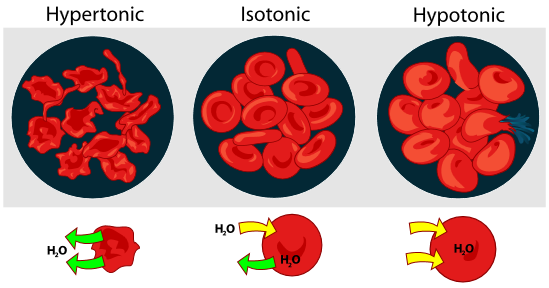## Diffusion

Diffusion is a transport phenomenon where particles move from a region in which they are highly concentrated to a region in which they are less concentrated. The speed at which these particles move is known as the rate of diffusion and is influenced by the following factors:

• Temperature: If the temperature increases, diffusion rate increases.

When temperature increases, the kinetic energy of the particles increases, which means that the particles move faster. Thus, particles spread faster in the solution and increase the rate of diffusion.

• Size of the particle: If particle size increases, rate of diffusion decreases.

Particles of larger size become less mobile in solvents, decreasing their speed and thus decreasing the diffusion rate.

• Mass of the particle: If the mass of the particle increases, the rate of diffusion decreases.

Like larger particles, heavier particles are less mobile in solution and decrease the rate of diffusion. In a solution, the solute is the substance that you want to dissolve and the solvent is the media that dissolves the solute. In the case of cells, water is the major constituent and it is the main solvent where substances are dissolved to be able to participate in reactions.

## Osmosis

Osmosis is a special form of diffusion where the water concentrations of two solutions separated by a partially permeable membrane reach equilibrium. This equilibration happens when water molecules move from the most concentrated solution to the least concentrated one.

In the case of cells, osmosis can occurs as cell membranes are semi-permeable. The following three situations may happen:

• Hypotonic medium -> the concentration of the solute outside the cell is lower than in the cell. Due to osmosis, there is a net movement of water molecules from outside the cell to the inside the cell, causing them to swell.

• Isotonic medium -> the solute outside the cell has the same concentration as inside the cell. Therefore, there is no net movement of water molecules across the cell membrane in any direction.

• Hypertonic medium -> the concentration of the solute outside the cell is higher than inside the cell. This leads to the net movement of water molecules from inside the cell to the medium outside, causing them to shrink.

Osmosis phenomena need to be controlled and are very important in the case of blood cells. If erythrocytes are surrounded by a hypotonic solution, there will be a net movement of water molecules into the cells which will cause them to swell and eventually burst. On the other hand, if cells are surrounded by a hypertonic solution, they will lose water and shrink.## Water potential

Water potential (ψ) quantifies the tendency of water to move from one region to another, such as in osmosis. It is the sum of several potentials including the pressure potential (ψp) and solute potential (ψπ).

ψ = ψp + ψπ

• Pressure potential (ψp): If pressure increases, water potential increases.

• Solute potential (ψπ): If solute concentration increases, water potential decreases.

The solute potential (ψπ) can be calculated with van't Hoff equation: ψπ = i∙C∙R∙T

Where i is the van 't Hoff factor or ionization constant (ratio of particles in solution to amount dissolved), C is the molar concentration of the solute (mol/L), R is the ideal gas constant (0.00831 L∙bar/mol∙K), and T is the absolute temperature (K). Notice that ionization constant is 1 for molecules that do not ionize when dissolved.